## TI-89 Tutorial

March 21, 2003
Updated on April 16, 2006The TI-89 is a great calculator, it's one of the best calculators right now (there's the HP-49G which is a good calculator too, the TI-92 Plus offer the same specifications as the TI-89 but offers Geometry possibilities with the Cabri Géomoètre and the Geometer's Sketchpad).

For the latest and most up-to-date tutorial download this tutorial in PDF format:TI-89 Tutorial

Top Solving equations & system of linear equations:
• Suppose we want to solve the following equation: -3x3 +3 x2 -2x+5=0
The syntax is :

solve(-3x^3+3x^2-2x+5=0,x)
or
zeros(-3x^3+3x^2-2x+5,x)I have written ",x" after the equation because the variable to solve for in this equation is x

• Suppose we want to solve the following system of equations:

```2x-3y+5z=-1 ``````-3x+5y-2z=3 ``````5x-7y+8z=-2 ```

solve(2x+3y+5z=-1 and -3x+5y-2z=3 and 5x-7y+8z=-2,{x,y,z})
or
zeros({2x+3y+5z+1 ,-3x+5y-2z-3 ,5x-7y+8z+2},{x,y,z})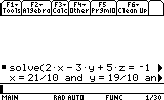Top Derivation & Integration:
• Derivation

Suppose we want to compute the derivative of : x2+3x-5
The syntax is

d(function,variable,degree)
degree can be omitted,it's 1 by default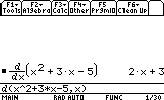Compute the partial derivative fx of f(x)=sin(x*y) +cos2(x+y)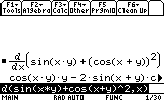• Integration:
Let's say we want to integrate the function sin(x):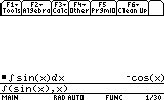Compute the double integral of : [x2 y + y2 +sin(y)] dxdy (we integrate for x supposing y constant then we integrate for y supposing x constant)Let's say we want to calculate the value of the integral of x*cos(x) between 1 and 10:Top Limits, sums & Taylor expansions
• Limits

Suppose we want to compute the limit of x2 when x tend to infinity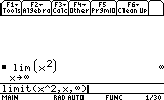The syntax is:

lim(function,variable,point,direction)
direction is both 1 and -1 and can be omitted
1: limit from right
2: limit from left

• Sum:

sum k2 for k between 1 and n• Taylor expansions:

The syntax for computing Taylor series is:

taylor(function,variable,degree,point)
point can be omitted , it's 0 by default

Suppose we want to know the 6th degree Taylor expansion of sin(x) around 0: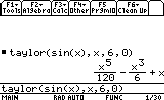Top Polynomials
• Expanding polynomials

The syntax is

expand(polynomial,variable)

Let's say we want to expand (x + y)4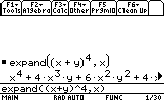• Factoring polynomials

The syntax is:

factor(function,variable)

Let's factor the function x2-9• Common denominator:

Let's put on the same denominator the function: (1/x2)+(1/(y2+1))Top Number operations:
• Factoring a number:

factor(number)

Let's factor the number 1050 for example: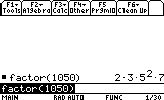• Finding the GCD (Great Commun Divisor) & LCM (Least Commun Multiple) of 2 numbers:

gcd(number1,number2)
lcm(number1,number2)

GCD & LCM of many numbers:
Let's find the GCD & LCM of 3 numbers:

gcd(gcd(number1,number2),number3)
lcm(lcm(number1,number2),number3)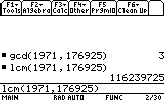• Testing if a number is prime or not

isPrime(number)

Let's see if 997 is prime or not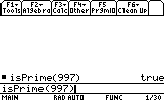• Finding the factorial of a number:

Let's find : 64!Top Differential Equations

Let's solve the following differential equation: x''+w2x=0

deSolve(function,x,y)
We must rename x to yNote that the result is: @3 cos (w.x)+@4 sin(w.x)
@3 and @4 are constants

Top Sequence

Suppose we want to find the terms of the following sequence: Un+1=2*Un+2 with U0=2
We can use 2 methods : the when function or by using the Sequence mode of the calculator.

1. when() function

• The syntax of this function is :

when(condition,true value,false value,unknown value)
false value & unknown value can be omitted.

when(n=0,2,2*u(n-1)+2) ->u(n)
The sign " -> " is to store the function in u(n)
To compute u, we write : u(1)Suppose we want to find the 5 first terms of the sequence, we should write:
{u(1),u(2),u(3),u(4),u(5)}2. Using the Sequence mode:

Let's take the previous example:
The syntax is:

u1=2*u1(n-1)+2
ui1=2
ui1 is the initial term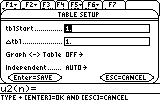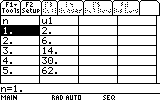Note that the table start at 1 so en u0 is equal to n=1 on the calculator ,there is a shift of 1 between the calculator and the real world.

Top